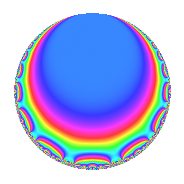# Properties

 Label 189.2.e.bLevel 189 Weight 2 Character orbit 189.e Analytic conductor 1.509 Analytic rank 0 Dimension 2 CM disc. -3 Inner twists 4

# Learn more about

## Newspace parameters

 Level: $$N$$ = $$189 = 3^{3} \cdot 7$$ Weight: $$k$$ = $$2$$ Character orbit: $$[\chi]$$ = 189.e (of order $$3$$ and degree $$2$$)

## Newform invariants

 Self dual: No Analytic conductor: $$1.5091725982$$ Analytic rank: $$0$$ Dimension: $$2$$ Coefficient field: $$\Q(\sqrt{-3})$$ Coefficient ring: $$\Z[a_1, \ldots, a_{7}]$$ Coefficient ring index: $$1$$ Sato-Tate group: $\mathrm{U}(1)[D_{3}]$

## $q$-expansion

Coefficients of the $$q$$-expansion are expressed in terms of a primitive root of unity $$\zeta_{6}$$. We also show the integral $$q$$-expansion of the trace form.

 $$f(q)$$ $$=$$ $$q$$ $$+ ( 2 - 2 \zeta_{6} ) q^{4}$$ $$+ ( 1 - 3 \zeta_{6} ) q^{7}$$ $$+O(q^{10})$$ $$q$$ $$+ ( 2 - 2 \zeta_{6} ) q^{4}$$ $$+ ( 1 - 3 \zeta_{6} ) q^{7}$$ $$+ 2 q^{13}$$ $$-4 \zeta_{6} q^{16}$$ $$+ 7 \zeta_{6} q^{19}$$ $$+ ( 5 - 5 \zeta_{6} ) q^{25}$$ $$+ ( -4 - 2 \zeta_{6} ) q^{28}$$ $$+ ( -11 + 11 \zeta_{6} ) q^{31}$$ $$+ 10 \zeta_{6} q^{37}$$ $$-13 q^{43}$$ $$+ ( -8 + 3 \zeta_{6} ) q^{49}$$ $$+ ( 4 - 4 \zeta_{6} ) q^{52}$$ $$+ 13 \zeta_{6} q^{61}$$ $$-8 q^{64}$$ $$+ ( 16 - 16 \zeta_{6} ) q^{67}$$ $$+ ( 7 - 7 \zeta_{6} ) q^{73}$$ $$+ 14 q^{76}$$ $$+ 4 \zeta_{6} q^{79}$$ $$+ ( 2 - 6 \zeta_{6} ) q^{91}$$ $$+ 5 q^{97}$$ $$+O(q^{100})$$ $$\operatorname{Tr}(f)(q)$$ $$=$$ $$2q$$ $$\mathstrut +\mathstrut 2q^{4}$$ $$\mathstrut -\mathstrut q^{7}$$ $$\mathstrut +\mathstrut O(q^{10})$$ $$2q$$ $$\mathstrut +\mathstrut 2q^{4}$$ $$\mathstrut -\mathstrut q^{7}$$ $$\mathstrut +\mathstrut 4q^{13}$$ $$\mathstrut -\mathstrut 4q^{16}$$ $$\mathstrut +\mathstrut 7q^{19}$$ $$\mathstrut +\mathstrut 5q^{25}$$ $$\mathstrut -\mathstrut 10q^{28}$$ $$\mathstrut -\mathstrut 11q^{31}$$ $$\mathstrut +\mathstrut 10q^{37}$$ $$\mathstrut -\mathstrut 26q^{43}$$ $$\mathstrut -\mathstrut 13q^{49}$$ $$\mathstrut +\mathstrut 4q^{52}$$ $$\mathstrut +\mathstrut 13q^{61}$$ $$\mathstrut -\mathstrut 16q^{64}$$ $$\mathstrut +\mathstrut 16q^{67}$$ $$\mathstrut +\mathstrut 7q^{73}$$ $$\mathstrut +\mathstrut 28q^{76}$$ $$\mathstrut +\mathstrut 4q^{79}$$ $$\mathstrut -\mathstrut 2q^{91}$$ $$\mathstrut +\mathstrut 10q^{97}$$ $$\mathstrut +\mathstrut O(q^{100})$$

## Character Values

We give the values of $$\chi$$ on generators for $$\left(\mathbb{Z}/189\mathbb{Z}\right)^\times$$.

 $$n$$ $$29$$ $$136$$ $$\chi(n)$$ $$1$$ $$-\zeta_{6}$$

## Embeddings

For each embedding $$\iota_m$$ of the coefficient field, the values $$\iota_m(a_n)$$ are shown below.

For more information on an embedded modular form you can click on its label.

Label $$\iota_m(\nu)$$ $$a_{2}$$ $$a_{3}$$ $$a_{4}$$ $$a_{5}$$ $$a_{6}$$ $$a_{7}$$ $$a_{8}$$ $$a_{9}$$ $$a_{10}$$
109.1
 0.5 + 0.866025i 0.5 − 0.866025i
0 0 1.00000 1.73205i 0 0 −0.500000 2.59808i 0 0 0
163.1 0 0 1.00000 + 1.73205i 0 0 −0.500000 + 2.59808i 0 0 0
 $$n$$: e.g. 2-40 or 990-1000 Significant digits: Format: Complex embeddings Normalized embeddings Satake parameters Satake angles

## Inner twists

Char. orbit Parity Mult. Self Twist Proved
1.a Even 1 trivial yes
3.b Odd 1 CM by $$\Q(\sqrt{-3})$$ yes
7.c Even 1 yes
21.h Odd 1 yes

## Hecke kernels

This newform can be constructed as the kernel of the linear operator $$T_{2}$$ acting on $$S_{2}^{\mathrm{new}}(189, [\chi])$$.